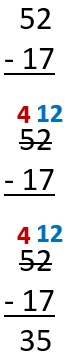# Math Worksheets Land

Math Worksheets For All Ages

# Math Worksheets Land

Math Worksheets For All Ages

Home > Math Topics > Subtraction >

# Mixed Number Subtraction

This section is meant to serve as a place to review your subtraction skills at all levels. We have many different places throughout our site that allow you to learn single-, double-, and triple-digit differences. We also have several sections that allow you to practice this operation with very large values or what is referred to as long subtraction. The goal of this section is to give you place to learn how to tackle problems that are all over the place. This mimics what you will see in the real world and help you master the use of this calculation. As you progress forward, you will see this skill in all different forms of mathematics from basic word problems all the way to and including calculus. This series of lessons and worksheets helps students learn to master subtraction skills.

### Aligned Standard: 2.NBT.B.7

• Answer Keys - These are for all the unlocked materials above.

### Homework Sheets

Mostly two and three digit subtraction, but pay attention there are a few curve balls.

• Homework 1 - Subtraction and addition are related.
• Homework 2 - Whatever you subtract from a number should create the starting number when added to the difference.
• Homework 3 - Add your final result to what you subtracted from the original number. You should get your starting number.

### Practice Worksheets

Straight up problems can be found in 1 and 2, but 3 is a matching set for you.

• Practice 1 - Single digit subtraction is very similar to counting backwards.
• Practice 2 - In this case we are facing a situation of subtraction based on place value.
• Practice 3 - Subtract the following and find the matches to the difference.

### Math Skill Quizzes

A long skill drill series for you.

• Quiz 1 - We always start by subtracting the lowest place value first (ones place).
• Quiz 2 - We start from the rightmost position i.e. ones place.
• Quiz 3 - How to do, smaller number – larger number, now? Borrow a ten from the tens place.

### How to Review SubtractionOut of the four basic arithmetic operations including addition, subtraction, multiplication, and division, the simplest are subtraction and addition. Not only is subtraction easy, but it is very simple verify the answer that one gets. This is because if you add the value that you have calculated to the subtrahend, you will get the minuend as the result. A quick reminder on the part of subtraction. This operation between the core components of the operation are termed as: Minuend - Subtrahend = Difference.

While teaching this skill can seem overwhelming at times, especially with students that do very little on their own outside of your classroom. This is just like teaching any other skill. When students might struggle give them something concrete to work with. If you create a systematic approach for students to use to tackle these problems, it becomes very doable for them. So, how do you proceed with subtraction? Consider an example: 52 - 17.Write it Vertically - The first thing you have to do is write the sum down vertically. When students present the problem in this manner, it makes the place values evident and clear to visualize.

Process It Right to Left - You need to understand that you always start from the right-hand side of the sum. Now, you see that 2 is less than 7 and you cannot subtract 7 from 2. What to do?

Borrow and Regroup - What you can do is borrow from the digit on the left-hand side(tens place). You can borrow one from 5 and reduce it to 4. The 1 you borrow from the 5, will convert 2 into 12 and you can easily subtract 7 from 12.

Fire Away - Now that you have each place of the minuend with values that are larger than all the place values of subtrahend, you can just process the simple operations. At the ones place: 12 - 7 = 5. At the tens place 4 - 1 = 3. The final answer will be 35.

Now you can easily verify your answer. You take the final answer and add it to the smaller number in the sum. The result will be equal to the larger number in the sum.

Unlock all the answers, worksheets, homework, tests and more!
Save Tons of Time! Make My Life Easier Now

## Thanks and Don't Forget To Tell Your Friends!

I would appreciate everyone letting me know if you find any errors. I'm getting a little older these days and my eyes are going. Please contact me, to let me know. I'll fix it ASAP.